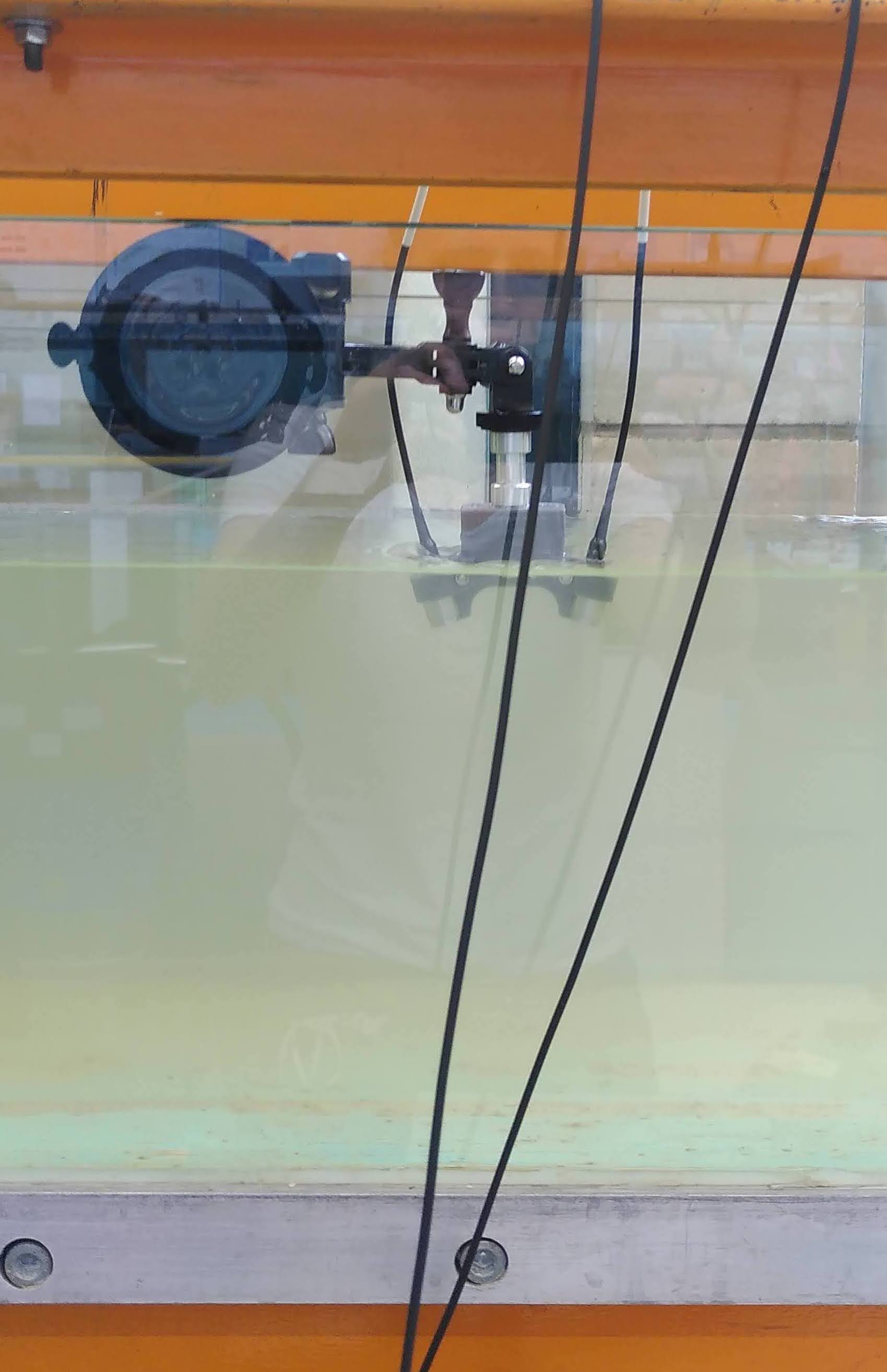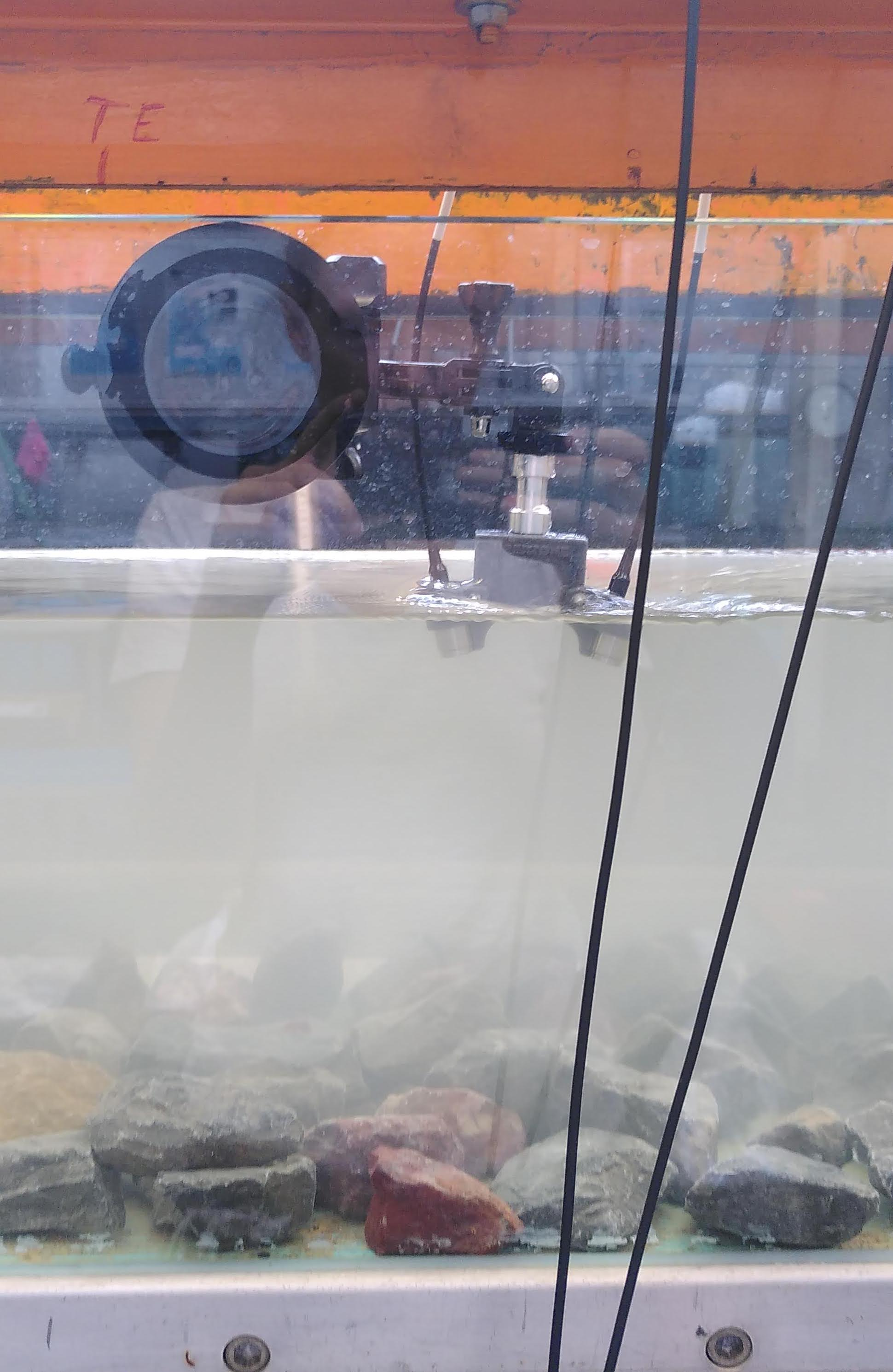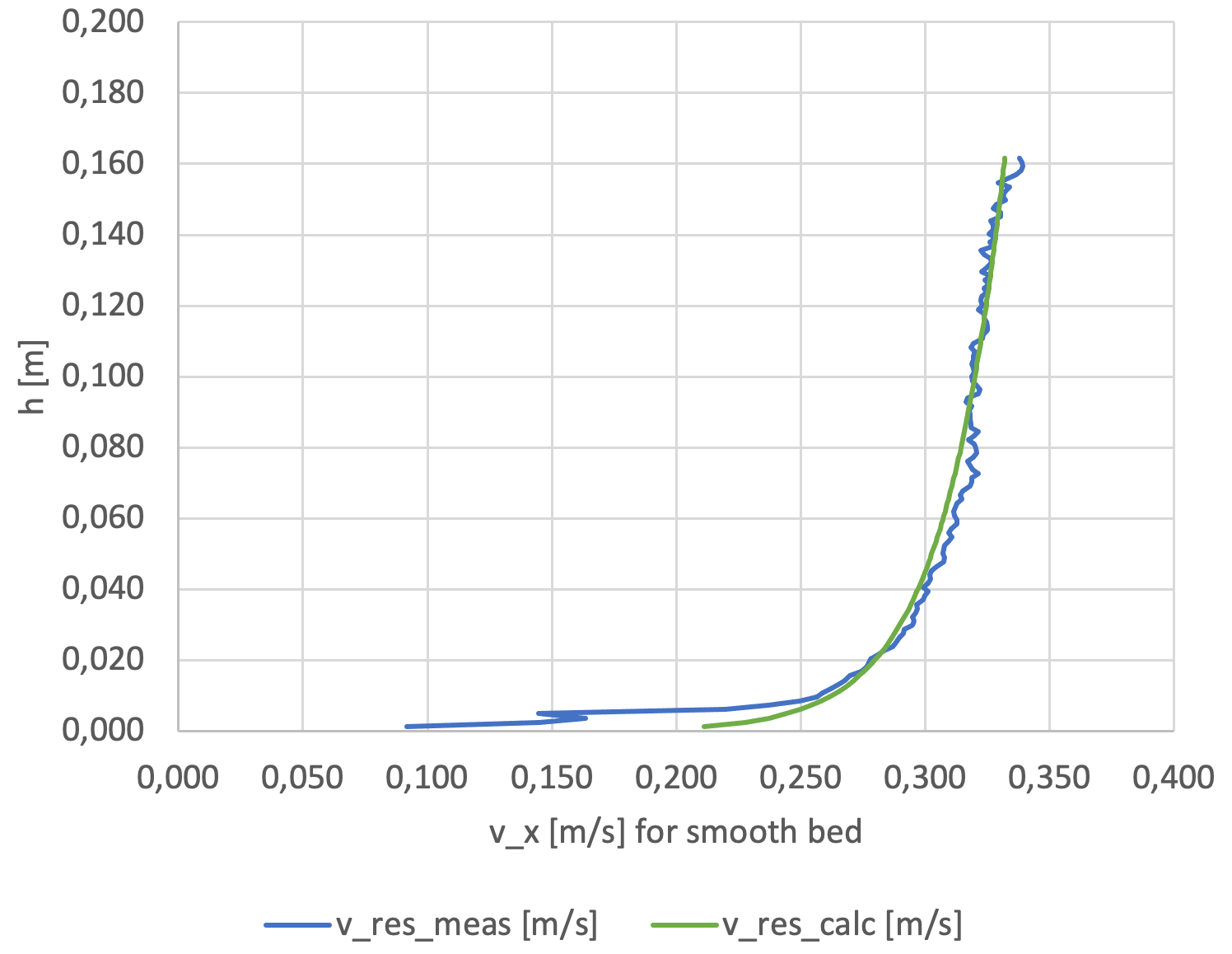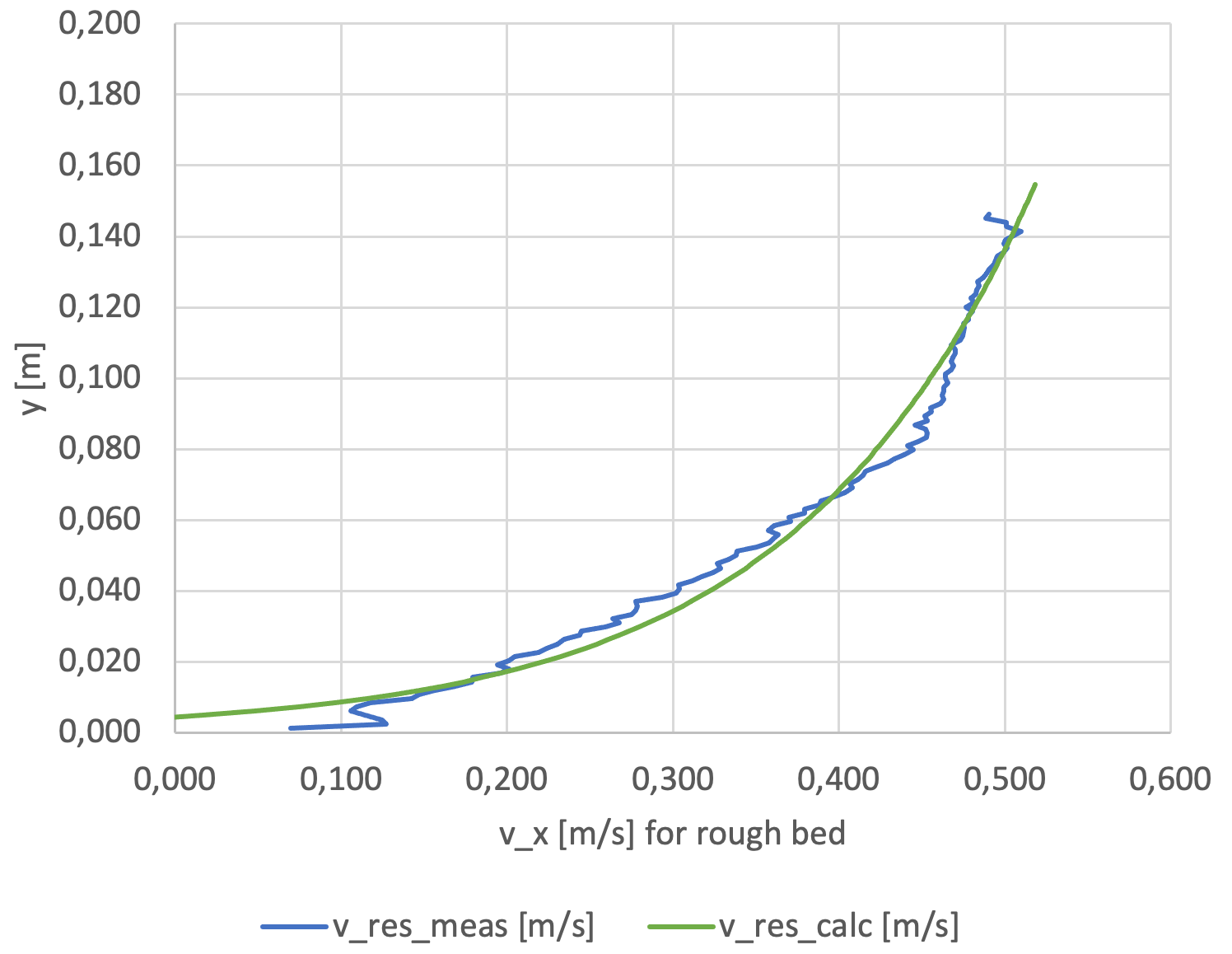### Velocity profile above smooth or rough bed

In a flume of the Technische Hochschule Karlsruhe (HKA), a part of the bed was covered with stones to create a rough bed.

Measurements of velocity profiles were done with a UB-Lab P and a pair of 3MHz transducers with a Doppler angle of 75° to the main flow axis (X, U). The transducer holder, with suction cup adaptor, was installed at the water surface to measure the vertical profile.

Doppler velocities of both transducers were filtered by the Doppler SNR (Signal to Noise Ratio) indicating the quality of the velocity estimation. The bottom of the flume was also detected. And those Doppler velocities were averaged and combined to get an average U,W velocity profile, see 2C monostatic velocity.

It was then possible to fit the profiles above rough vs smooth bed with a roughness model:

$${{u(z)} \over {u^*}} = {{1} \over {\kappa}} ⋅ ln \left( {{h(z)} \over {k_s}} \right) + C$$with:

• $$u(z)$$: velocity at height z,
• $$u^*$$: shear velocity $${u^*} = \sqrt { \tau_0 \over \rho }$$,
• $$\tau_0$$: shear stress $$\tau_0 = \rho⋅g⋅h⋅I$$,
• $$I$$: slope,
• $$\kappa$$: Karman constant $$\kappa = 0.41$$,
• $$h$$: total water depth,
• $$h(z)$$: water depth at height z,
• $$C$$: constant.These measurements were done on the occasion of the WUD 2022 workshop.